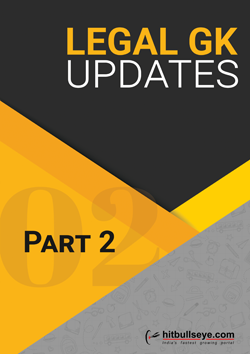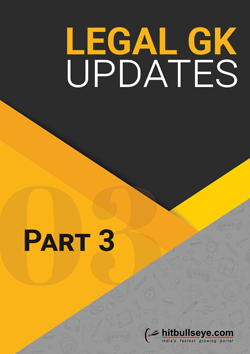Crack the SLAT Analytical Reasoning Section

In this article, we are going to provide you with tips and techniques required to crack the SLAT analytical reasoning section of the entrance examination. After reading this article, you will be familiar with:
• the areas from which the questions usually appear in SLAT analytical reasoning section,
• the approach for getting expertise in these areas,
• followed by some actual questions of the exam along with its solutions and the learning from those questions.SLAT Analytical Reasoning Important Topics:
This section, named as SLAT analytical reasoning has a significant portion of questions from the quantitative aptitude or Mathematics, as it is popularly called. The major areas covered in this examination are
• Series-Numeric and Alphabetic
• Coding and Decoding
• Blood Relations
• Clocks and Calendars
• Ratio and Mixtures
• Permutation, Combination and Probability
• Syllogism
• Time & Work
• Basic algebra
• Cause and Effect questions
• Percentage and Profit & Loss
• Critical Reasoning
• Misc reasoning
The exam has around 15 questions from Clocks, Calendars, Series, Coding-decoding and blood relations only. From all the other areas, the paper has 2-3 questions in the exam. Needless to say, you need to revise all the basics of mathematics with special focus on these areas. Do practice sufficient number of questions from these areas, in order to become proficient in SLAT analytical reasoning section.
Suggested Action :
Refine your preparation by taking FREE SET Mock Tests
1. Clear basics of all Topics:
The questions on SLAT analytical reasoning section are of "elementary" nature, so the foremost thing is to clear your basics. Most of the questions require only the basic understanding of the concepts and hence will give you easy scoring options.
The questions, which have appeared in the last few years, will give you a better and clearer idea about the kind of questions and the difficulty level of SLAT analytical reasoning section.
Illustration 1: The mother is four times older than her son. After four years the sum of their age would be 63 years. What is her son's present age?
1. 12
2. 13
3. 11
4. 12.5
Sol: Let the age of the mother be M and that of the son be S. The two equations would be M = 4S...eq (i) and (M + 4) + (S + 4) = 63 ... eq (ii). You can solve these two equations and get the answer. Besides that you can solve it with logic as well i.e. If the sum of their ages after four years will be 63 years, at present they would be younger by 4 years each. So the sum of their present ages would be 63-8 = 55 years. The ratio of their ages is given as 4 : 1. Hence the age of the son will be 55 × 1/5 = 11 years.
Learning: In this question, you learn that it is not necessary to make equations for ratio or ages based questions, you can solve that question logically as well. As in this case, when the age after/before a certain year is given, the present sum of their ages can be found by working logically. Otherwise it is also advisable to find the answer by working with the help of options in case of questions based on ages.
Illustration 2: What is the probability of drawing a king and a queen one after the other without replacement from a well-shuffled deck of cards?
1. 8/169
2. 4/663
3. 1/169
4. 4/51
Sol: The question can be solved logically. The king can be drawn in 4/52 ways and now the remaining cards are 51 as it is without replacement and there are 4 queens. The queen can be drawn in 4/51 ways. Hence the answer to the question will be (4/52) * (4/51) = 4/663.
Learning: The topic of probability is not extremely technical as most of the students consider it to be. The basic questions like this and others, which usually appear in the competitive papers, can be solved by applying the simple counting principal only. Make it a point to solve the seemingly mathematical problems by way of simple logic. Do consider it as a puzzle instead of treating it as mathematics.
2. Speed & Accuracy:
Your focus should be on solving the questions with a good speed without compromising on the accuracy. If your fundamentals are clear, you can solve questions in a limited time.
3. Practice, Practice & Practice:
Preparing for SLAT requires consistency, dedication, focus and good mentorship. Plan your preparation keeping in mind the time you have and your areas of strength & weakness. It is also essential that you take multiple Mock Tests and solve previous years' papers.
Suggested Action :
This section is named as analytical reasoning, and the kind of questions it contains clearly indicate that even the examiner wants you to apply your mind analytically to answer these questions. Do not think it is mathematics, which you cannot solve, rather it contains logic based real life problems, which need to be dealt with application of mind. Do revise the above-mentioned areas and practice sufficient number of questions from each area.
Key Learning:
• Practicing SLAT mock tests and SLAT previous year's papers help in mastering your preparation.
• Past year's paper analysis shows that all the areas in analytical reasoning section are important, therefore, you should not leave any topic untouched.
• Basic understanding of all topics and sufficient practice is required to score well in SLAT reasoning section.
If you have any question, suggestion or feedback, feel free to post it in the discussion box given below